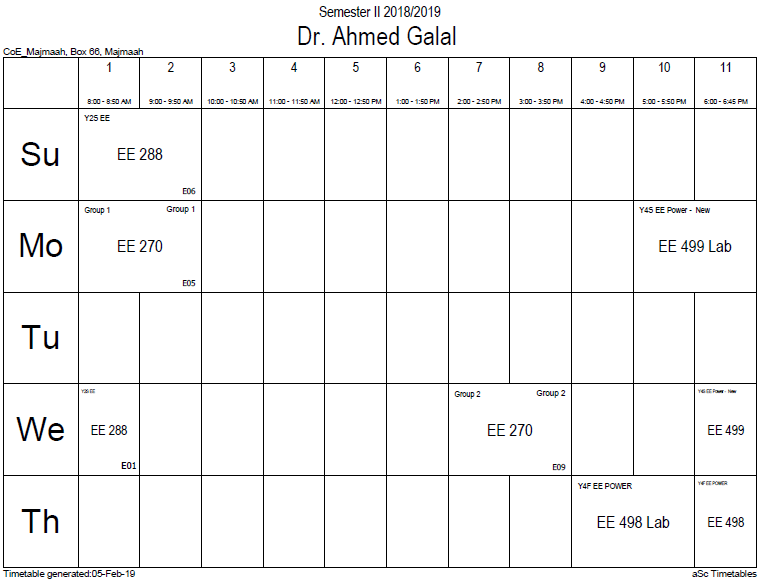## displacement field

In physics, the electric displacement field, denoted as$mathbf{D}$, is a vector field that appears in Maxwell's equations. It accounts for the effects of free charge within materials. "D" stands for "displacement," as in the related concept of displacement current in dielectrics. In free space, the electric displacement field is equivalent to flux density, a concept that lends understanding to Gauss's law.

In a dielectric material the presence of an electric field E causes the bound charges in the material (atomic nuclei and their electrons) to slightly separate, inducing a local electric dipole moment. The electric displacement field D is defined as$mathbf{D} equiv varepsilon_{0} mathbf{E} + mathbf{P},$

where$varepsilon_{0}$ is the vacuum permittivity (also called permittivity of free space), and P is the (macroscopic) density of the permanent and induced electric dipole moments in the material, called the polarization density. Separating the total volume charge density into free and bound charges:$ho = ho_f + ho_b$

the density can be rewritten as a function of the polarization P:$ho = ho_f - ablacdotmathbf{P}.$

P is a vector field whose divergence yields the density of bound charges$ho_b$ in the material. The electric field satisfies the equation:$ablacdotmathbf{E} = frac{1}{varepsilon_0} ( ho_f + ho_b) = frac{1}{varepsilon_0}( ho_f - ablacdotmathbf{P})$

and hence$ablacdot (varepsilon_0mathbf{E} + mathbf{P}) = ho_f$.

The displacement field therefore satisfies Gauss's law in a dielectric:$ablacdotmathbf{D} = ho - ho_b = ho_f$ .

D is not determined exclusively by the free charge. Consider the relationship:$abla imes mathbf{D} = varepsilon_{0}( abla imes mathbf{E}) + ( abla imes mathbf{P})$

Which, by the fact that E has a curl of zero in electrostatic situations, evaluates to:$abla imes mathbf{D} = abla imes mathbf{P}$

Which can be immediately seen in the case of some object with a "frozen in" polarization like a bar electret, the electric analogue to a bar magnet. There is no free charge in such a material, but the inherent polarization gives rise to an electric field. If the wayward student were to assume the D field were entirely determined by the free charge, he or she would immediately conclude the electric field were zero in such a material, but this is patently not true. The electric field can be properly determined by using the above relation along with other boundary conditions on the polarization density yielding the bound charges, which will, in turn, yield the electric field.

In a linear, homogeneous, isotropic dielectric with instantaneous response to changes in the electric field, P depends linearly on the electric field,$mathbf{P} = varepsilon_{0} chi mathbf{E},$

where the constant of proportionality$chi$ is called the electric susceptibility of the material. Thus$mathbf{D} = varepsilon_{0} (1+chi) mathbf{E} = varepsilon mathbf{E}$

where$varepsilon = varepsilon_{0} varepsilon_{r}$ is the permittivity, and$varepsilon_{r} = 1 + chi$ the relative permittivity of the material.

In linear, homogeneous, isotropic media$varepsilon$ is a constant. However, in linear anisotropic media it is a matrix, and in nonhomogeneous media it is a function of position inside the medium. It may also depend upon the electric field (nonlinear materials) and have a time dependent response. Explicit time dependence can arise if the materials are physically moving or changing in time (e.g. reflections off a moving interface give rise to Doppler shifts). A different form of time dependence can arise in a time-invariant medium, in that there can be a time delay between the imposition of the electric field and the resulting polarization of the material. In this case, P is a convolution of the impulse response susceptibility χ and the electric field E. Such a convolution takes on a simpler form in the frequency domain—by Fourier transforming the relationship and applying the convolution theorem, one obtains the following relation for a linear time-invariant medium:$mathbf{D(omega)} = varepsilon (omega) mathbf{E}(omega) ,$

where$omega$ is frequency of the applied field (e.g. in radian/s). The constraint of causality leads to the Kramers–Kronig relations, which place limitations upon the form of the frequency dependence. The phenomenon of a frequency-dependent permittivity is an example of material dispersion. In fact, all physical materials have some material dispersion because they cannot respond instantaneously to applied fields, but for many problems (those concerned with a narrow enough bandwidth) the frequency-dependence of$varepsilon$; can be neglected.

At a boundary,$D_{1,perp} - D_{2,perp} = sigma_f$, where$sigma_f$ is the free charge density.

### Office HoursNo office hours### My Timetable### Contactsemail: [email protected]

Phone: 2570

### Welcome

Welcome To Faculty of Engineering### IEEEhttp://www.ieee.org/

/

### Bookmarkshttp://www.utk.edu/research/

http://science.doe.gov/grants/index.asp

http://www1.eere.energy.gov/vehiclesandfuels/

http://www.eere.energy.gov/

### Upcoming Conferences### Engineering quotes### Travel Web Siteshttp://www.hotels.com/

http://www.orbitz.com/

http://www.hotwire.com/us/index.jsp

http://www.kayak.com/

### Blackboardستقام اختبارات الميدتيرم يوم الثلاثاء 26-6-1440

حسب الجدول المعلن بلوحات الاعلان

### Summer trainingThe registration for summer training will start from 5th week of second semesterClass registration week 1

### برنامج التجسير### إحصائية الموقع

عدد الصفحات: 2879

البحوث والمحاضرات: 1280

الزيارات: 99456